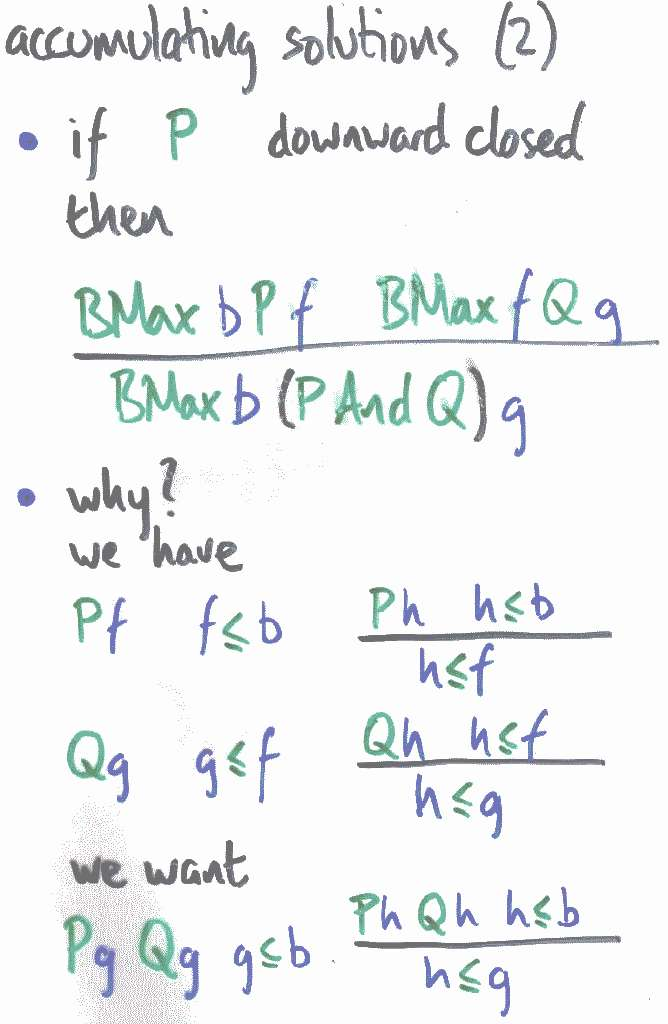Next: the optimist's proof | Prev: downward closure | Up: contentsThe optimist's theorem says that if we can decompose a problem into a downward-closed bit P and the rest (not necessarily downward-closed) Q, then we may perform relative optimisation in sequence, reducing the accumulated bound b to some f which maximises P, then maximising Q relative to f. Effectively, we reduce the P component of the constraint to bounding by f.

Decomposing the hypotheses and the conclusion, we acquire or require three things each:

• satisfaction of the property
• bounding by the accumulator
• optimality of the solution

You can tell this is an abstract slide, because it's all green and blue, not red and purple.

Let's complete the proof.

Next: the optimist's proof | Prev: downward closure | Up: contents

Conor's Work Page